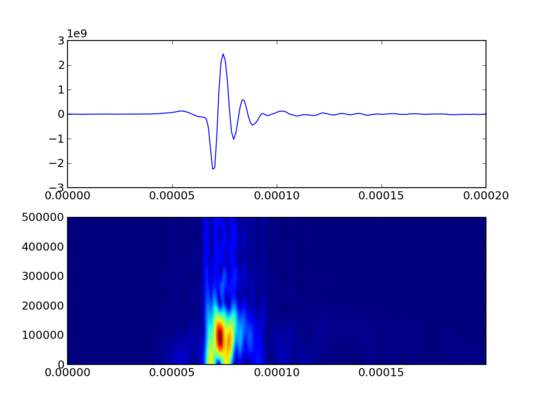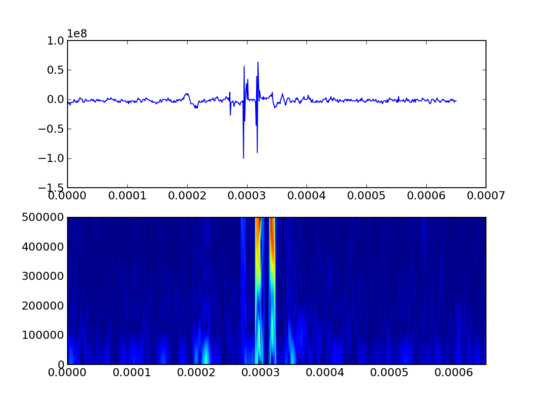# Python and the STFT

I’ve been going through biosonar data and while the SciPy specgram method is serviceable, I was interested in a short-time Fourier transform (STFT) implementation. There are a couple of ad hoc routines on Stack Overflow and the like, but I’ve started off with the Google Code PyTFD module. There are others out there as well, at least two projects including an STFT implementation are aimed at extracting time and frequency data from musical recordings. I may have a look at one or both of those at some point.

In any case, installing PyTFD involves downloading the code via Subversion and then running the setup.py script.

Since I spent more time than I think was absolutely necessary getting a couple of examples done with the STFT, let me run through an example in the hopes that helps somebody.

[python]
# Imports
from __future__ import division
from pytfd.stft import *
from pytfd import windows

import numpy as np
import numpy.fft as nf
import matplotlib
matplotlib.use(‘Agg’)
import scipy
import scipy.signal as spsig
import pylab
from pylab import *

# […]

w = windows.rectangular(8)
Y_stft = stft(clkdata,w)
extt = [0,Y_stft.shape*1e-6,0,5e5]
pylab.imshow(abs(Y_stft)[Y_stft.__len__()//2:],
extent=extt,
aspect=”auto”,
origin=”upper”)
[/python]

OK, so there’s a fair amount of things to be imported along the way. The first three items (lines 2 to 4) are specifically for setting up access to PyTFD’s STFT method. Line 18 sets up the window function to use in the STFT. Line 19 actually does the work, getting the resulting multidimensional Numpy array with the STFT result given a Numpy array input and the window.
Line 20 sets up the extent array to express the size of the X range and the Y range covered by the STFT. Lines 20 to 24 puts the result in a subplot. There are some issues there. The STFT results are essentially a whole series of Fourier transforms, and those have both negative and positive frequencies, and are complex values to boot. So the “abs” function provides a magnitude for each point. The slice yields just the positive frequency range. Then the extent gets set to the range represented by the STFT. The “aspect” parameter is set to “auto” so that the X and Y ranges can be calculated separately by Matplotlib. The “origin” is set to “upper” to put the frequencies in the expected orientation.

Here’s a couple of the outputs:#### Wesley R. Elsberry

Falconer. Interdisciplinary researcher: biology and computer science. Data scientist in real estate and econometrics. Blogger. Speaker. Photographer. Husband. Christian. Activist.

### One thought on “Python and the STFT”

•2012/04/01 at 6:43 am